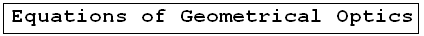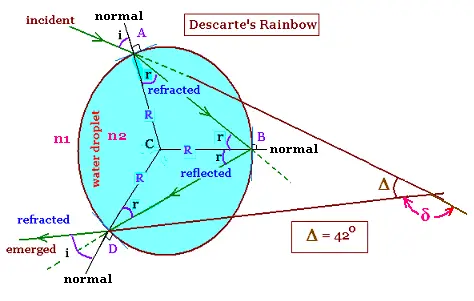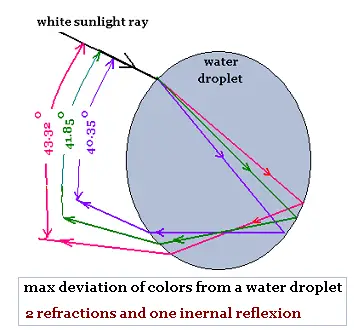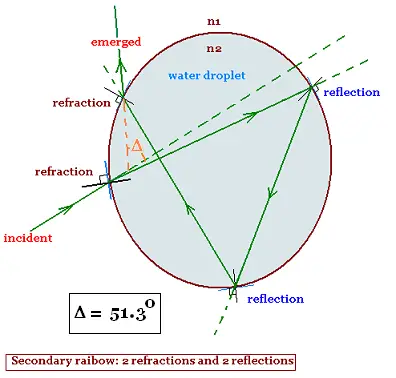ÂGeometrical Optics: Geometrical Optics & Physics Optics. a Huygens Principle: Wavefronts. a Reflection: The first law of Geometrical Optics. a Refraction: The second law of Geometrical Optics: Snell's law. a Critical angle: Internal total reflection. a images formed by reflection Spherical concave mirrors. Mirror equation. a images formed by refraction Spherical convex mirrors .. a Fermat's principle: Fermat's principle for reflection and refraction.. a lenses: Thin lenses and Lens-makers' equation .. a prism: Prism, colors minimum deviation .. a Human eye: near point, far point, nearsightedness, farsightedness.. a Microscope: Microscope: simple, compound, and magnification.. a Telescope: Telescope: magnification.. a Resolution: optical microscope, electron microscope .. a dispersion: dispersion, more about the refractive index, and colors of a prism .. a parallel sheet: deviation by a parallel sheet .. a thin films : thin films and Newton's rings.. a Newton's rings: thin films and Newton's rings.. a Descartes rainbow: Primary and secondary rainbows .. a Some applications More fun with Optics .. a Optics calculator: Optics calculator: all the related calculations .. a home The fundamental, and just this .. a scientificSentence a __________________ a

Descartes' Rainbow
Optics - Â  Â  Geometrical Optics..

### 1. Descartes' RainbowThe white ray light travels from air of index of refraction n1, through a water droplet of index of refraction n2, and emerges to the air toward an observer.
What are the colors could we see? What is the minimum deviation of the ray?. We will use the model of Descartes to answer these two questions. We will see the two rainbows: the primary (one internal reflection and two refractions) and the secondary (two internal reflections and two refractions).

Â Â  Î´ is the deviation between the incidence and emergence of the sun light ray.
Î´ = (i - r) + (2Ï - 2r) + (i - r)
= 2Ï + 2i - 4r

We have Î = 2Ï - Î´
Î = 2Ï - (2Ï + 2i - 4r)

Î = 4r - 2i Â Â Â (1)

d(Î)/di = d(4r - 2i)/di = 0
Then: dr/di = 1/2 Â Â Â (2)
From Snell's law, we have:
n1 sin i = n2 sin r â sin r = (n1/n2) sin i. The ratio n1/n2 for air-water is generally taken equal to 3/4. Therefore:
r = arcsin((n1/n2) sin i)
dr/di = [1 - (n1/n2)2 sin2 i]-1/2 x (n1/n2) cos i Â Â Â (3)
The relationship (2) becomes:
1/2 = [1 - (n1/n2)2 sin2 i]-1/2 x (n1/n2) cos i
Or
(1/4) [1 - (n1/n2)2 sin2 i] = (n1/n2)2 cos2 i = (n1/n2)2 (1 - sin2 i)
Then:
(1/4) = (1/4) (n1/n2)2 sin2 i + (n1/n2)2 (1 - sin2 i) = (1/4) (n1/n2)2 sin2 i + (n1/n2)2 - (n1/n2)2 sin2 i
Thus:
(1/4) - (n1/n2)2 = - (3/4) (n1/n2)2 sin2 i
or
(n1/n2)2 - (1/4) = (3/4) (n1/n2)2 sin2 i (4/3) [(n1/n2)2 - 1/4] (n2/n1)2 = sin2 i (4/3) [1 - (1/4) (n2/n1)2] = sin2 i
sin i = ((4/3) [1 - (1/4) (n2/n1)2])1/2
sin i = ((1/3) [4 - (n2/n1)2])1/2
i = arcsin [([4 - (n2/n1)2]/3)1/2] Â Â Â (4)

i = arcsin [([4 - (n2/n1)2]/3)1/2]

for n2/n1 = 4/3, we have :
i = arcsin [((1/3) [4 - 16/9])1/2] = arcsin((20/27)1/2)= 59.40

i = 59.40 o

From Snell's law: n1 sin i = n2 sin r, we have:
r = arcsin[(n1/n2) sin i] = arcsin[(3/4) (20/27)1/2] = arcsin[0.64] = 40.20 = 40.20 o

r = arcsin[(n1/n2) sin i]

The relationship (1) becomes:
Î = 4r - 2i

Î = 4r - 2i = 4(40.20)o - 2(59.40)o = 160.80o - 118.80 o = 42o.

Î = 42o
Then, the deviation is : Î´ = 2Ï - Î´ = 222 o
Note &delta is min, then Î is max.

The relative index of refraction of a ray from the menium n1 to the medium n2 is:
n = n2/n1

Thus, Snell's law is rewritten, if i and r are the angles od incidence and refraction respectively, as:
sin i = n sinr â sin r = (1/n) sin i
Using the relationship (2): dr/di = 1/2, we get:
cos r (dr/di) = (1/2) cos r = (1/n) cos i
Thus cos i = (n/2) cos r = (n/2) [1 - sin2r]1/2 = (n/2) [1 - (1/n)2 sin2i]1/2
= (n/2) [1 - (1/n)2 (1 - cos2i)]1/2 = (n/2) [1 - (1/n)2 + (1/n)2 cos 2i]1/2
Then:
cos2 i = (n/2)2 [1 - (1/n)2 + (1/n)2 cos2i] =
(n/2)2 - (1/4)+ (1/4) cos2i)
Or:
(3/4) cos2i = (n/2)2 - (1/4). Hence
3 cos2i = n2 - 1. Then cos i = [(n2 - 1)/3]1/2

cos i = [(n2 - 1)/3]1/2

### 2. Properties of Descartes' RainbowHere is a table that gives the color, the wavelength in vacuum, the related index of refraction in water, and the related Descartes rainbow max deviation.
The index of refraction of air is taken equal to 1.0003 for all these wavelengths of the white light ray spectrum.

 color wavelength index of refraction max rainbow deviation violet 380450 1.3455 40.35 blue 450475 1.3425 40.77 green 495570 1.3350 41.85 yellow 570590 1.3334 42.08 orange 590620 1.3330 42.14 red 620750 1.3250 43.32

The values of the refractive indexes in water, in this table, are the average values taken from the results of philip Laven .

### 3. Secondary rainbowIn this case have, we have two internal reflections and two refractions:
Î´ = (i - r) + (2Ï - 2r) + (i - r) + 2Ï - 2r

Î´ = 2i - 6r + 4Ï
The condition of minimum is written as:
dÎ´/di = 0 = 2 - 6 (dr/di). Hence:
dr/di = 1/3

Using Snell's law:
sin r = (1/n) sin i, we get:
cos r (dr/di) = (1/3) cos r = (1/n) cos i
Thus cos i = (n/3) cos r = (n/3) [1 - sin2r]1/2 = (n/3) [1 - (1/n)2 sin2i]1/2
= (n/3) [1 - (1/n)2 (1 - cos2i)]1/2 = (n/3) [1 - (1/n)2 + (1/n)2 cos 2i]1/2
Then:
cos2 i = (n/3)2 [1 - (1/n)2 + (1/n)2 cos2i] = (n/3)2 - (1/9)+ (1/9) cos2i = 0. Or:
(8/9) cos2i = (n/3)2 - (1/9). Hence
8 cos2i = n2 - 1. Then cos i = [(n2 - 1)/8]1/2.

The condition of minimum is:
cos i = [(n2 - 1)/8]1/2

For the example of n = 4/3, we have:
i = arcos [(n2 - 1)/8]1/2 = arcos [(16/9 - 1)/8]1/2 = 72.0 o

i = 72.0 o

r = arcsin [(1/n) sin i] = arcsin [(3/4) sin 72.0] = 45.45o
r = arcsin [(1/n) sin i] = arcsin [(3/4) sin 72.0] = 45.45o

r = 45.45o

Î´ = 2i - 6r + 4Ï = 2 x 72.0 - 6 x 45.45 + 00 = 144 - 272.7 + 720 = 591.3 == 591.3 - 360 = 231.3

Î = Î´ - Ï = 231.3 - 180 = 51.3o

Î = 51.3o

Â

 @import url(http://www.google.com/cse/api/branding.css);Custom Search Â© 2009. The scientificsentence . All rights reserved.